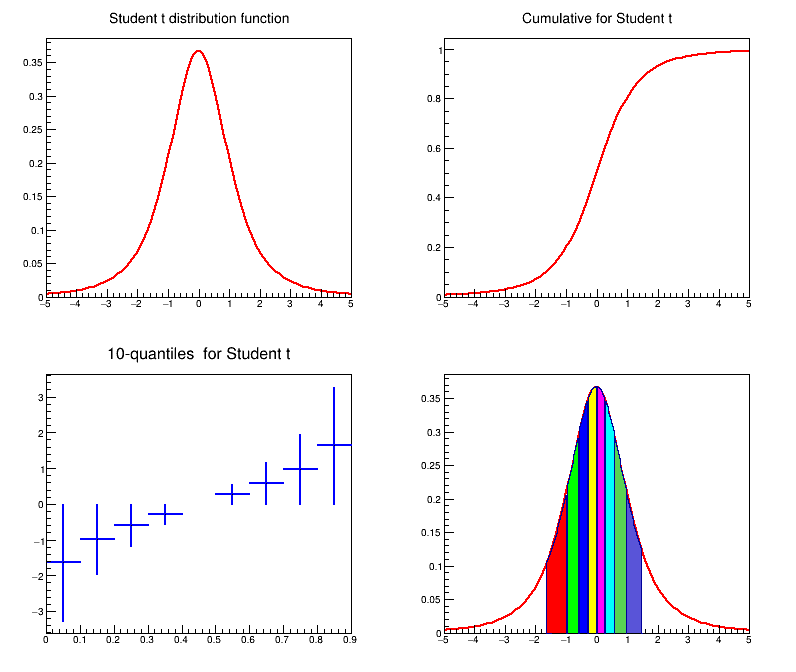# tStudent¶

Example macro describing the student t distribution

root: .x tStudent.C


It draws the pdf, the cdf and then 10 quantiles of the t Student distribution

Author: Magdalena Slawinska
This notebook tutorial was automatically generated with ROOTBOOK-izer from the macro found in the ROOT repository on Wednesday, August 17, 2022 at 09:34 AM.

In :
/* gSystem->Load("libMathMore");*/


this is the way to force load of MathMore in Cling

In :
ROOT::Math::MathMoreLibrary::Load();

int n=100;
double a=-5.;
double b=5.;

Info in <ROOT::Math::MathMoreLibrary>: libMathMore has been loaded.


double r = 3;

In :
TF1* pdf = new TF1("pdf", "ROOT::Math::tdistribution_pdf(x,3.0)", a,b);
TF1* cum = new TF1("cum", "ROOT::Math::tdistribution_cdf(x,3.0)", a,b);

TH1D* quant = new TH1D("quant", "", 9, 0, 0.9);

for(int i=1; i < 10; i++)
quant->Fill((i-0.5)/10.0, ROOT::Math::tdistribution_quantile((1.0*i)/10, 3.0 ) );

double xx;
xx = -1.5;
for(int i=1; i<9; i++)
xx[i]= quant->GetBinContent(i);
xx = 1.5;
TH1D* pdfq;


int nbin = n/10.0;

In :
for(int i=0; i < 9; i++) {
int nbin = n * (xx[i+1]-xx[i])/3.0 + 1.0;
TString name = "pdf";
name += i;
pdfq[i]= new TH1D(name, "", nbin,xx[i],xx[i+1] );
for(int j=1; j<nbin; j++) {
double x= j*(xx[i+1]-xx[i])/nbin + xx[i];
pdfq[i]->SetBinContent(j, ROOT::Math::tdistribution_pdf(x,3));
}
}

TCanvas *Canvas = new TCanvas("DistCanvas", "Student Distribution graphs", 10, 10, 800, 700);
pdf->SetTitle("Student t distribution function");
cum->SetTitle("Cumulative for Student t");
quant->SetTitle("10-quantiles  for Student t");
Canvas->Divide(2, 2);
Canvas->cd(1);
pdf->SetLineWidth(2);
pdf->DrawCopy();
Canvas->cd(2);
cum->SetLineWidth(2);
cum->SetLineColor(kRed);
cum->Draw();
Canvas->cd(3);
quant->Draw();
quant->SetLineWidth(2);
quant->SetLineColor(kBlue);
quant->SetStats(0);
Canvas->cd(4);
pdfq->SetTitle("Student t & its quantiles");
pdf->SetTitle("");
pdf->Draw();

Warning in <TH1::TH1>: nbins is <=0 - set to nbins = 1


pdfq->SetAxisRange(-1.5, 0, 1.5,1.0);

In :
pdfq->SetTitle("Student t & its quantiles");
for(int i=0; i < 9; i++) {
pdfq[i]->SetStats(0);
pdfq[i]->SetFillColor(i+1);
pdfq[i]->Draw("same");
}
Canvas->Modified();
Canvas->cd();


Draw all canvases

In :
gROOT->GetListOfCanvases()->Draw()